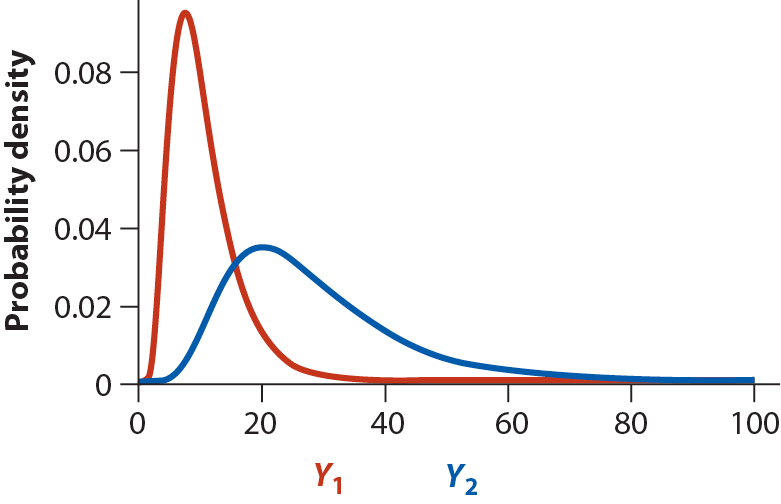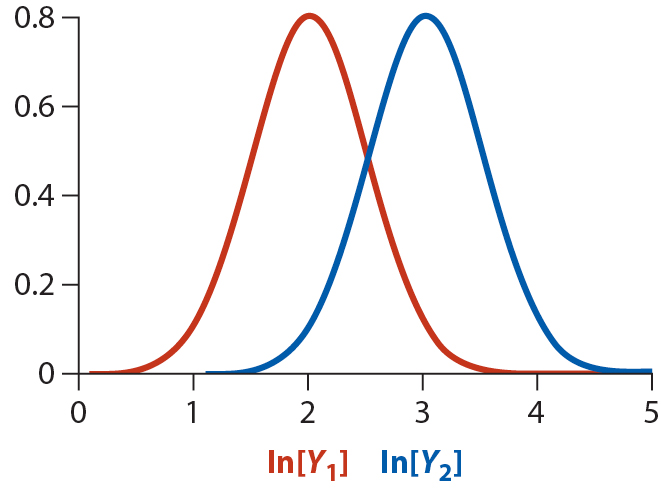Handling violations of assumptions (cont'd)

M. Drew LaMar
April 4, 2016Data transformations

• Ignore the violations of assumptions
• Transform the data
• Use a nonparametric method
• Use a permutation test (computer-intensive methods)

Definition: A data transformation changes each measurement by the same mathematical formula.

Data transformations

Common transformations:

• Log transformation (data skewed right) $Y^{\prime} = \ln[Y]$
• Arcsine transformation (data are proportions) $p^{\prime} = \arcsin[\sqrt{p}]$
• Square-root transformation (data are counts) $Y^{\prime} = \sqrt{Y + 1/2}$

Data transformations

Other transformations:

• Square transformation (data skewed left) $Y^{\prime} = Y^2$
• Antilog transformation (data skewed left) $Y^{\prime} = e^{Y}$
• Reciprocal transformation (data skewed right) $Y^{\prime} = \frac{1}{Y}$
• Box-Cox transformation (skew) $Y^{\prime}_{\lambda} = \frac{Y^{\lambda} - 1}{\lambda}$

Log transformations - When to use

• Measurements are ratios or products
• Frequency distribution is skewed right
• Group having larger mean also has larger standard deviation
• Data span several orders of magnitude

Log transformations - When to use

• Measurements are ratios or products
• Frequency distribution is skewed right
• Group having larger mean also has larger standard deviation
• Data span several orders of magnitudeLog transformations - When to use

• Measurements are ratios or products
• Frequency distribution is skewed right
• Group having larger mean also has larger standard deviation
• Data span several orders of magnitudeLog transformations - How to useHypothesis testing

shapiro.test(log(marine$biomassRatio)) Shapiro-Wilk normality test data: log(marine$biomassRatio)